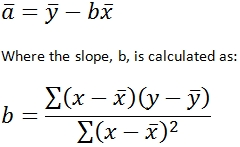# SQL Server INTERCEPT function

INTERCEPT

Updated: 24 September 2010

Note: This documentation is for the SQL2008 (and later) version of this XLeratorDB function, it is not compatible with SQL Server 2005.
Click here for the SQL2005 version of the INTERCEPT function

Use the multi-input aggregate INTERCEPT function to calculate the point at which a line will intersect the y-axis by using existing x-values and y-values. The y-intercept is the value of the point which intersects the line at x = 0. In linear equations that are in the slope intercept form of y = mx + b, the value of b is the y-intercept. The equation for intercept is:Syntax
SELECT [wctStatistics].[wct].[INTERCEPT] (
,<@Known_y, float,>
,<@Known_x, float,>)
Arguments

@Known_y
the y-values to be used in the INTERCEPT calculation. @Known_y is an expression of type float or of a type that can be implicitly converted to float.
@Known_x
the x-values to be used in the INTERCEPT calculation. @Known_x is an expression of type float or of a type that can be implicitly converted to float.
Return Types
float
Remarks
·         INTERCEPT is an AGGREGATE function and follows the same conventions as all other AGGREGATE functions in SQL Server.
Examples
In this example, we calculate the intercept for a single set of x- and y-values
SELECT wct.INTERCEPT(y, x) as INTERCEPT
FROM (
SELECT 0.75, 1 UNION ALL
SELECT 2.5, 2 UNION ALL
SELECT 6.75, 3 UNION ALL
SELECT 10, 4
) n(x,y)

This produces the following result
INTERCEPT
----------------------
0.972553699284009

(1 row(s) affected)
In this example, we will populate some temporary table with some historical financial information and then calculate the intercept. First, create the table and put some data in it:
CREATE TABLE #c(
SYM         NVARCHAR(5),
YE          BIGINT,
REV         FLOAT,
GPROF       FLOAT,
OPINC       FLOAT,
NETINC      FLOAT
)

INSERT INTO #c VALUES('YHOO',2009,6460.32,3588.57,386.69,597.99)
INSERT INTO #c VALUES('YHOO',20,72.5,4185.14,12.96,418.92)
INSERT INTO #c VALUES('YHOO',2007,6969.27,4130.52,695.41,639.16)
INSERT INTO #c VALUES('YHOO',2006,6425.68,3749.96,940.97,751.39)
INSERT INTO #c VALUES('YHOO',2005,5257.67,3161.47,1107.73,1896.23)
INSERT INTO #c VALUES('GOOG',2009,23650.56,14806.45,8312.19,6520.45)
INSERT INTO #c VALUES('GOOG',20,21795.55,13174.04,5537.21,4226.86)
INSERT INTO #c VALUES('GOOG',2007,16593.99,9944.9,54.44,4203.72)
INSERT INTO #c VALUES('GOOG',2006,10604.92,6379.89,3550,3077.45)
INSERT INTO #c VALUES('GOOG',2005,6138.56,3561.47,2017.28,1465.4)
INSERT INTO #c VALUES('MSFT',2010,62484,509,24167,18760)
INSERT INTO #c VALUES('MSFT',2009,58437,46282,21225,14569)
INSERT INTO #c VALUES('MSFT',20,60420,48822,22271,17681)
INSERT INTO #c VALUES('MSFT',2007,51122,40429,18438,14065)
INSERT INTO #c VALUES('MSFT',2006,44282,36632,16064,12599)
INSERT INTO #c VALUES('ORCL',2010,26820,21056,9062,6135)
INSERT INTO #c VALUES('ORCL',2009,23252,18458,8321,5593)
INSERT INTO #c VALUES('ORCL',20,22430,17449,7844,5521)
INSERT INTO #c VALUES('ORCL',2007,17996,13805,5974,4274)
INSERT INTO #c VALUES('ORCL',2006,14380,11145,4736,3381)
INSERT INTO #c VALUES('SAP',2009,10672,6980,2588,1748)
INSERT INTO #c VALUES('SAP',20,11575,7370,2701,1847)
INSERT INTO #c VALUES('SAP',2007,10256,6631,2698,1906)
INSERT INTO #c VALUES('SAP',2006,9393,6064,2578,1871)
INSERT INTO #c VALUES('SAP',2005,8509,5460,2337,1496)
In this example, we will calculate the intercept of the operating income (OPINC) against the revenue (REV)
SELECT SYM
,wct.INTERCEPT(OPINC,REV) as INTERCEPT
FROM #c
GROUP BY SYM
This produces the following result.
SYM   INTERCEPT
----- ----------------------
GOOG 168.902505528265
MSFT -2828.427434343
ORCL -474.4613098912
SAP   1510.83759005268
YHOO 3520.06698156002

(5 row(s) affected)
Let’s say we wanted to perform the same analysis as above, but we only want to return the results where the slope is positive.
SELECT SYM
,wct.INTERCEPT(OPINC,REV) as INTERCEPT
FROM #c
GROUP BY SYM
HAVING wct.INTERCEPT(OPINC,REV) > 0
This produces the following result.
SYM   INTERCEPT
----- ----------------------
GOOG 168.902505528265
SAP  1510.83759005268
YHOO 3520.06698156002

(3 row(s) affected)Copyright 2008-2019 Westclintech LLC         Privacy Policy        Terms of Service